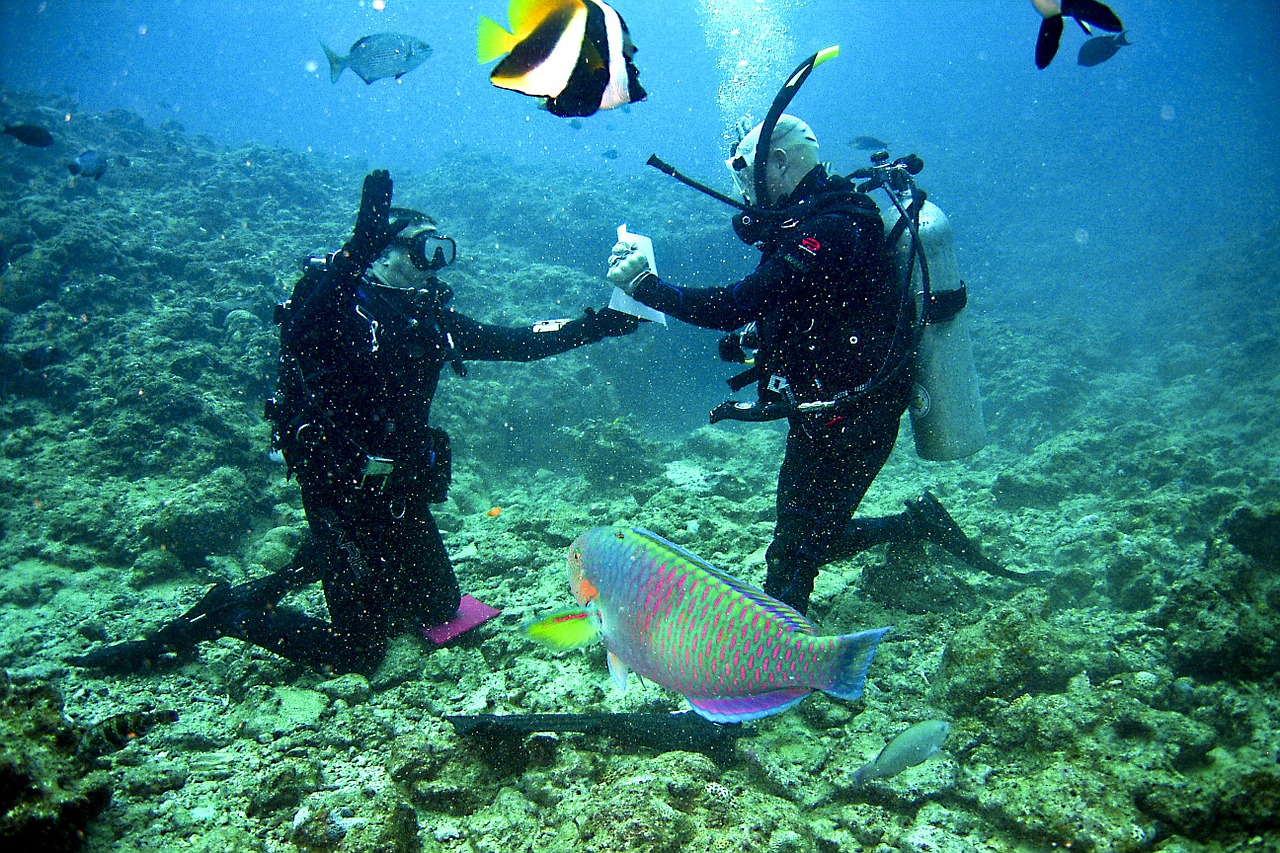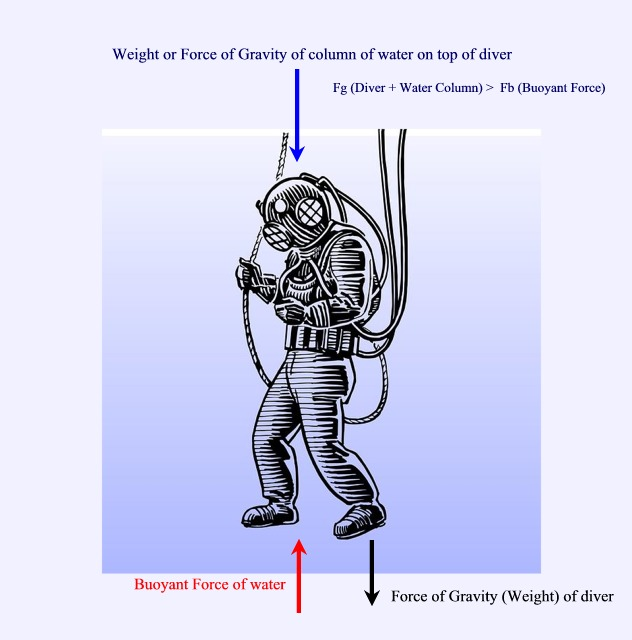#### Pressure in Liquids

Pressure in liquids is also known as hydrostatic pressure.

As stated in a previous lesson, as the depth of a column of liquid or gas increases, the pressure also increases.

Therefore an object at the bottom of a tank of a liquid will experience more pressure than at the top of the tank.

Scuba divers experience greater pressures as they go deeper.This is due to the fact that the weight (or force of gravity) of the overlying fluid on top of the object also increases.

Consider the deep-sea diver below.The buyoant force acting upwards on him is much smaller than the combined Forces of Gravity acting on him and the Force of gravity of the column of water on top of him.  It also depends on the density of the the liquid.

We can therefore generalize this concept by relating the above factors in  a simple equation

Pressure in a liquid (P) = Density (D) x Depth (h) x Gravitational Constant (g)

g = 9.8 N/Kg

P = D x h x g

Example:

What is the pressure experienced by a 140 Kg diver at a depth of 20 m in Lake Ontario?  Assume fresh water density of 1.0 Kg/m3

Note that the mass of the diver is not involved in this calculations. Why not?

Given:

• D Water = 1.0 Kg/m3
• h = 20 m
• g = 9.8 N/Kg

Solution:

P = D x h x g

= 1.0 Kg/m3 x 20 m x 9.9 N/Kg

= 196.0 N/m2

= 196 kPa